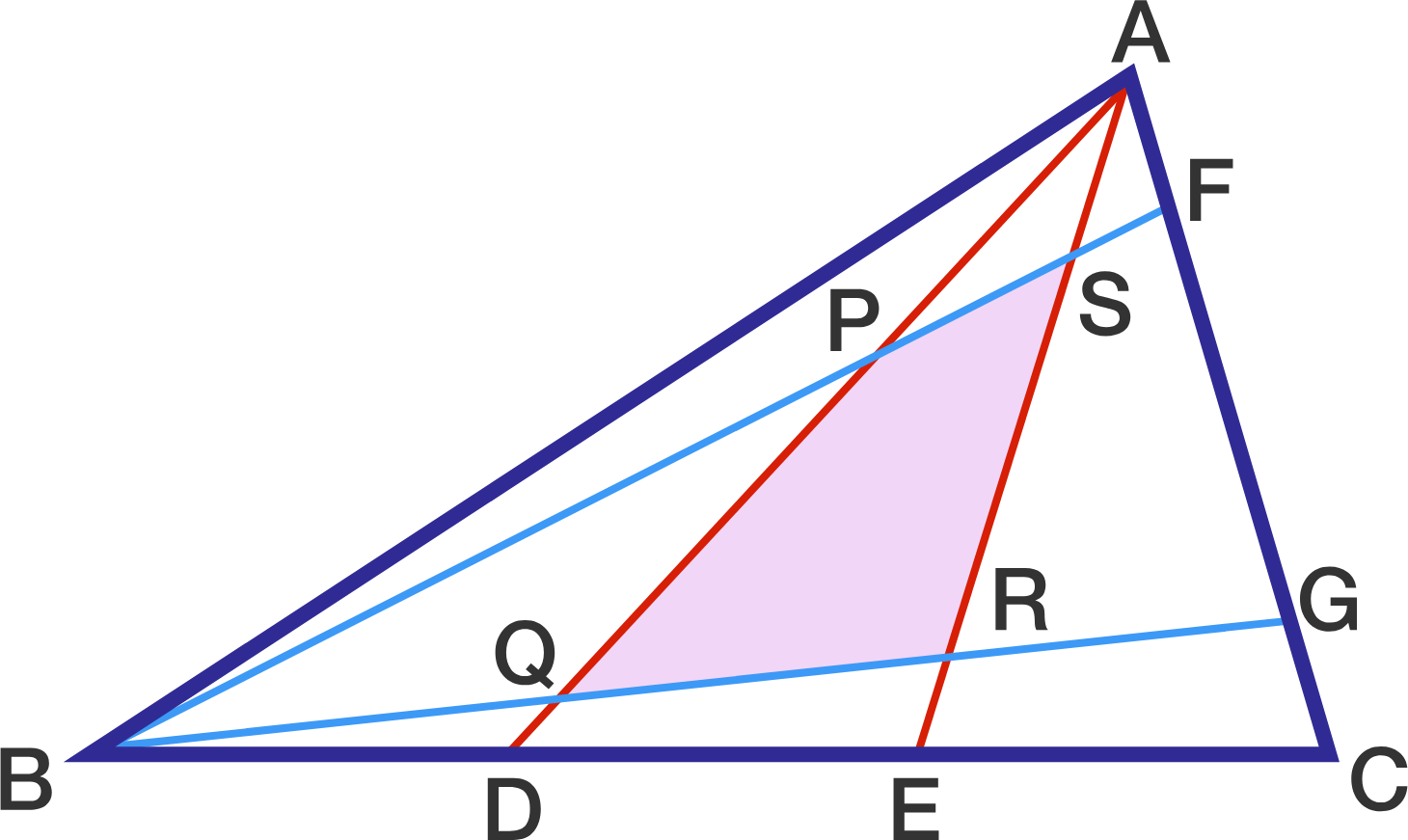Geometry Level 5In a triangle $ABC$, points $D$ and $E$ are on $BC$ such that $| BD | : | DE | : | EC | = 1 : 1:1$, and points $F$ and $G$ are on $AC$ such that $| AF | : | FG | : |GC | = 1 : 3 : 1$.

The quadrilateral $PQRS$ is formed by the intersection of segments $BF, BG, AD$ and $AE$.

If the area of the triangle $ABC$ is 1001 square units, what is the area of quadrilateral $PQRS$?

×